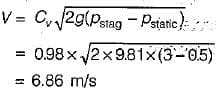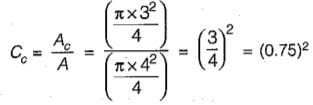# Test: Energy Equation & Its Applications - 1

## 10 Questions MCQ Test GATE Civil Engineering (CE) 2023 Mock Test Series | Test: Energy Equation & Its Applications - 1

Description
Attempt Test: Energy Equation & Its Applications - 1 | 10 questions in 30 minutes | Mock test for Civil Engineering (CE) preparation | Free important questions MCQ to study GATE Civil Engineering (CE) 2023 Mock Test Series for Civil Engineering (CE) Exam | Download free PDF with solutions
QUESTION: 1

### A Pitot-static tube, with a coefficient of velocity 0.98 is used to measure the velocity of water in a pipe. The stagnation pressure recorded is 3 m and the static pressure is 0.5 m. What is the velocity of flow?

Solution:QUESTION: 2

Solution:
QUESTION: 3

### A flow through an expanding tube at a constant rate is called

Solution:

Flow of liquid through a long pipe of constant diameter at a constant rate is steady uniform flow; flow of liquid through a long pipe line of constant diameter, at either increasing or decreasing rate is unsteady-uniform flow; flow of liquid through a tapering pipe at a constant rate is steady-nonuniform flow and flow through a tapering pipe at either increasing or decreasing rate is unsteady- non-uniform flow.

QUESTION: 4

To avoid the tendency of separation of flow at the throat in a Venturimeter, the ratio of the diameter at the throat to the diameter of the pipe should be

Solution:

The ratio of throat to inlet diameters d2/d1 may range between 0.75 and 0.25, but the most commonly used ratio is 0.50.
A smaller ratio gives a higher difference in the piezometric heads (between the inlet and the throat) which can be measured more accuratedly by a differential manometer. At the same time, a smaller throat (that is a lower d2/d1 ratio) will mean higher throat velocities which may cause pressures low enough to liberate dissolved gases thereby creating conditions for cavitation to set in.

QUESTION: 5

Venturimeter is used to measure

Solution:

A venturi meter is a device which is used for measuring the rate of flow of fluid through a pipe. The basic principle on which a venturi meter works in that by reducing the cross-sectional area of the flow passage, a pressure difference is created and the measurement of the pressure difference enables the determination of the discharge through the pipe.

QUESTION: 6

A pipe of length more than double the diameter of the orifice fitted internally or externally to the orifice is called

Solution:
QUESTION: 7

A point in a compressible flow where the velocity of fluid is zero, is called

Solution:

At vena-contracta velocity of fluid is maximum at stagnation point velocity is zero and all kinetic energy is converted to pressure energy.

QUESTION: 8

The equation P/w + V2/2g + Z = constant is based on the following assumptions regarding the flow of fluid:

Solution:

The equation is Bernoulli’s equation. It is based on following assumptions:
(ii) fluid is incompressible
(iii) fluid is. non-viscous since viscous force has been neglected; and
(iv) it is applicable to points along a streamline

QUESTION: 9

A fluid jet discharging from a 4 cm diameter orifice has a diameter 3 cm at its vena contracta. If the coefficient of velocity is 0.98, the coefficient of discharge for the orifice will be

Solution:

For an orifice the coefficient of discharge Cd, coefficient of velocity Cv and coefficient of contraction Cc is related as,
Cd = Cc x CvCd = (0.75)2 x 0.98

QUESTION: 10

The coefficient of velocity for an orifice is given by (using usual notation)

Solution:Use Code STAYHOME200 and get INR 200 additional OFF Use Coupon Code